# Creating a 2D physics engine from scratch in Javascript

Writing a physics engine is an extremely complex task. It requires knowledge of physics, math and programming in order to create an accurate and efficient implementation. However, if we put aside performance and restrict us to the most important features, creating a simple engine is surprisingly straightforward and very satisfying.

In this article, we will implement a toy 2d physics engine that supports basic forces, collisions and constraints (joints) for circle-shaped objects. We will also implement a basic renderer for the engine using p5js, because what use is a physics engine if we can’t see what’s happening?

We’ll build stuff incrementally from the ground up, and interactive demos will be provided as we go along. This post is heavily inspired by the following Pezzza’s video which I encourage you to watch. I found it very interesting and I’ll follow it closely, so it provides a nice overview of what you’re going to see next. I suggest taking a look at his entire channel too because all the videos are awesome!

Now let’s start!

## Setting things up

The first step is to define the basic structure of our engine and rendering “pipeline”.

From what we know at this stage, we can image our engine has to hold a list of bodies that constitute the world. It’ll also need to expose some methods to create and manipulate them. For now, since we’re limiting ourselves to circle-shaped bodies, we just need to define an array to hold the bodies and one method to instantiate a circle:

```.wp-block-code {
border: 0;
}

.wp-block-code > span {
display: block;
overflow: auto;
}

.shcb-language {
border: 0;
clip: rect(1px, 1px, 1px, 1px);
-webkit-clip-path: inset(50%);
clip-path: inset(50%);
height: 1px;
margin: -1px;
overflow: hidden;
position: absolute;
width: 1px;
word-wrap: normal;
word-break: normal;
}

.hljs {
box-sizing: border-box;
}

.hljs.shcb-code-table {
display: table;
width: 100%;
}

.hljs.shcb-code-table > .shcb-loc {
color: inherit;
display: table-row;
width: 100%;
}

.hljs.shcb-code-table .shcb-loc > span {
display: table-cell;
}

.wp-block-code code.hljs:not(.shcb-wrap-lines) {
white-space: pre;
}

.wp-block-code code.hljs.shcb-wrap-lines {
white-space: pre-wrap;
}

.hljs.shcb-line-numbers {
border-spacing: 0;
counter-reset: line;
}

.hljs.shcb-line-numbers > .shcb-loc {
counter-increment: line;
}

.hljs.shcb-line-numbers .shcb-loc > span {
}

.hljs.shcb-line-numbers .shcb-loc::before {
border-right: 1px solid #ddd;
content: counter(line);
display: table-cell;
text-align: right;
-webkit-user-select: none;
-moz-user-select: none;
-ms-user-select: none;
user-select: none;
white-space: nowrap;
width: 1%;
}
```var Engine = function() {
return {
"bodies" : [],
"createCircle" : function(radius, x, y, options) {
let newCircle = {
"position": new Vector(x, y),
"previousPosition" : new Vector(x, y),
"acceleration" : new Vector(0, 0),
"color" : "#FF0000",
...options
};
this.bodies.push(newCircle);
return newCircle;
},
};
}```Code language: JavaScript (javascript)```

Each circle, or rather “body” as I will be referring to them generically from now on, has three fields that we use to handle the physics: `position`, `previousPosition` and `acceleration`. These would be common fields across all shape types, if we ever add something that’s not a circle.

There are then parameters specific to the body shape, like `radius` in this case. If we ever want to create other shapes we would need to change these accordingly, for example using `width` and `height` for a rectangle, or a list of vertices for more complex polygons.

We also set the body’s color, which, although it doesn’t pertain to the physics, is very convenient to have here to use in our rendering later.

Lastly, we append all the other key-value pairs passed as the `options` argument; this is useful to set additional parameters that we might implement later (e.g. if a body is fixed) or to override default values, for example for the color.

You might’ve noticed that we use a `Vector` class in the previous code. It’s a pretty simple class used to represent and manipulate 2D vectors and it’s defined in the utils.js file, along with some other constants.

Let’s now set up a p5js sketch that will work as our simple rendering pipeline. We’ll need this to visually test the code we’ve written.

``````/**
* Canvas width and height in pixels
*/
const WIDTH = 500;
const HEIGHT = 500;

let engine = Engine();

function setup() {
noStroke();
let c = createCanvas(WIDTH, HEIGHT);

c.mousePressed(function () {
});
}

/**
* This method is the main rendering loop of p5js and gets called to draw every frame.
* We reset the background and then just use p5js to draw a circle for each body, according
* to its parameters (color).
*/
function draw() {
background(255);

for (let body of engine.bodies) {
fill(body.color);
}
}
```Code language: JavaScript (javascript)```

For now we’re just looping over the bodies and drawing them using their fill color. When the mouse is clicked we ask the engine to create a new body at the mouse position.

The result is the following. Click anywhere inside the canvas to spawn a red ball.

## Verlet integration

Our basics are ready and we can now put in some magic. Let’s add Verlet integration into the mix!

If you opened the Wikipedia page you might’ve seen a lot of scary formulas. Fortunately, we don’t need to understand the full derivation in order to create a working implementation. We’re just interested in the final result, which is a method to compute an approximation for the motion of an object at discrete intervals of time.

In particular, if we have a body with position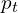, velocity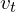and accelerationat time, the Verlet method allows us to compute the value of its position at the next time step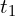, after an amount of time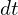has elapsed, that is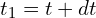. We can use this method iteratively to compute the trajectory of any object if we know the initial values of its position, velocity and acceleration.

This method is very simple to implement as the expressions for these variables are rather intuitive. Having defined the velocityas the difference between the current and the previous positions: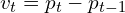the next position is given by: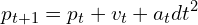If you are interested, the video at the start of the post goes more in depth on how to derive this result, but we can also just take this for granted. It’ll look like this when translated into code:

``````var bodyUpdate = function(dt) {
let velocity = this.position.sum(this.previousPosition.mult(-1));
this.previousPosition = this.position;
this.position = this.position.sum(velocity).sum(this.acceleration.mult(dt * dt));
this.acceleration = new Vector(0, 0);
}```Code language: JavaScript (javascript)```

The method takes care of updating both the previous and current position fields by using the formulas we just saw, taking the elapsed time `dt` as input. It will be attached to the body objects so the `this` in the example refers to an instance of a body. Notice that we reset the acceleration to 0 at the end of every update: this means that when we need to have a constant acceleration on a body we’ll need to apply it at every time step.

The method we just saw needs to be called for every body in the world, so we will create a `step(dt)` method inside our engine that does that:

``````"step" : function(dt) {
for (let body of this.bodies) {
body.update(dt);
}
},```Code language: JavaScript (javascript)```

We just created the main method of our engine, which advances the simulation by an interval of time `dt`. At the moment it just updates the bodies, but there’ll be more stuff to update later.

Finally, we need to call `step` at every frame and to do that is has to be inside the p5js `draw` method, since this works as our main loop. But the step method needs to know how much time has passed since the last iteration (the `dt` parameter) so we need to add some code to compute it. This is how our new `draw` method looks like:

``````// Set the starting time in milliseconds
var lastUpdate = Date.now();

function draw() {
background(255);

for (let body of engine.bodies) {
fill(body.color);
}

let delta = (Date.now() - lastUpdate) / 1000;
engine.step(delta);
lastUpdate = Date.now();
}
```Code language: JavaScript (javascript)```

Everything is ready to go, but as of now there’s no acceleration acting on the bodies so they’re not going to move just yet. Let’s add gravity by setting it inside the `step` method, with the addition of one line:

``````"step" : function(dt) {
for (let body of this.bodies) {
body.acceleration = new Vector(0, 200);
body.update(dt);
}
},```Code language: JavaScript (javascript)```

Notice that we need to apply the gravity at each step, because as we saw earlier the acceleration gets reset to 0 with every `bodyUpdate` call.

The acceleration has to be positive if we want objects to go downwards, because we’re using p5js and its frame of reference has the y axis going down. Also, the value of 200 comes because we are directly using pixels as our measurement unit.

Normally this is not a good idea, because the physics engine should be independent from the rendering pipeline. The ideal solution would be to make the engine use standard units like meters and then having the rendering pipeline decide how many pixels a meter corresponds to. In this case, for simplicity, we’re avoiding all of this and making the engine use pixels directly, which is equivalent of operating under the assumption that 1 pixel is equal to 1 meter.

Here’s the result:

The bodies will now fall out of the canvas.

## Constraints

Since we don’t want bodies to disappear into the void, we’ll enclose them inside a circular region that covers most of the canvas. Since the engine need to know the canvas size now, we’ll need these as parameters to our `Engine` function:

``````var Engine = function(width, height) {
return {
"width" : width,
"height" : height,
"bodies" : [],
//...,
//...,
};
};```Code language: JavaScript (javascript)```

Next we’ll need an `applyConstraints` method that’s called at every step and checks if part of the body is outside of the constraint. If that’s the case, we move the body the minimum amount needed to to bring it back inside.

Note that checking if a body is outside the constraint is particularly easy to do only because all of our bodies, as well as our constraint, are circles. It can get very tricky to do this for arbitrary shapes, but we don’t have to worry about that for now.

``````"applyConstraints" : function() {
/**
* this.width and this.height here refer to the canvas
*/
let radius = this.width / 2 * 0.95;
let center = new Vector(this.width / 2, this.height / 2);

for (let body of this.bodies) {
let diff = body.position.sum(center.mult(-1));
let dist = diff.length();

body.position = center.sum(t);
}
}
}```Code language: JavaScript (javascript)```

In order to understand how the code works, have a look at the following picture.

The red circle is currently outside the constraint. We can detect this because the length of the blue vector called `diff` in the code (i.e. the distance of the body from the center) it’s greater than the maximum allowed of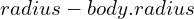. We need to move the object in the correct position, the one shown as a dotted circle.

This position will be exactly at a distance offrom the center, along the same direction as the `diff` vector. Basically we can obtain it by just scaling down the `diff` vector by the right amount. An easy way to do this is to normalize it and then multiply it byto bring it to the desired length. The sum of the result with the center is the correct position for the body.

The constraint isn’t a standard body, so it wouldn’t normally get rendered. For this reason, the code running here also has slight modifications to the rendering part, which I won’t bother showing here, to render the white circle on black background.

Notice how Verlet integration keeps working seamlessly and produces a plausible behaviour when interacting with the constraint!

## Collisions

As one might imagine, the mechanism to handle collisions is very similar to the one we just created for the constraints. Indeed, a collision is just a constraint that says that two bodies cannot overlap.

``````"checkCollisions" : function() {
for (let i = 0; i < this.bodies.length; i++) {
for (let k = 0; k < this.bodies.length; k++) {
if (i == k) continue;

let bodyA = this.bodies[i];
let bodyB = this.bodies[k];

let diff = bodyA.position.sum(bodyB.position.mult(-1));
let dist = diff.length();

let t = diff.mult(1 / dist);
bodyA.position = bodyA.position.sum(t.mult(0.5 * delta));
bodyB.position = bodyB.position.sum(t.mult(-0.5 * delta));
}
}
}
}```Code language: JavaScript (javascript)```

The handling is slightly different because we now move both bodies along the collision axis, while in the previous case the main constraint has a fixed position so we can only reposition the moving body.

Performance is very important for a solid physics engine. In this example, we’re using a naivealgorithm that checks all possible body pairs for collisions. This is highly inefficient because most of these bodies will be very distant from each other and we’re wasting computational power on checking collisions that can’t occur. Nevertheless, it seem to work well enough that we can be happy with it for the sake of this article. It should hopefully be able to handle a few thousands bodies even in the browser.

## Fixed bodies

At the moment all of our bodies are dynamic, so they react to forces and move accordingly. It’s often the case that we need to have the opposite, for example to create obstacles that don’t fall with gravity. These are called fixed bodies and are pretty easy to implement. They will be very useful in our next step when creating joints.

Since we can pass additional parameters with the `options` argument when creating a body, we’ll establish a boolean parameter `fixed` that defines the behaviour of the body. We then need to update our engine code accordingly, in order to avoid moving any object whose `fixed` is true. This involves, for example, not applying gravity and not moving the body on a collision: in general, every time we modify the position of a body we should avoid doing that if the body is fixed.

Fixed bodies will be rendered as gray in the following demos.

## Joints

A joint is a constraint that connects two (or more) bodies and limits their possible positions relative to each other.

There are a variety of joint types that a full-fledged physics engine can support, but since we’re clearly aiming for simplicity we’ll limit ourselves to the most basic distance joints.

As the name says, a distance joint regulates the distance between two bodies. The simplest implementation means that two bodies will have a fixed distance, which is what we’re going to do. A more advanced version can allow the user to set a minimum or maximum distance, rather than a fixed one.

Enforcing a fixed distance between two bodies is yet another slight variation of what we implemented for collisions and for the global constraint. For this reason, we’ll use the same exact technique to implement it.

To accommodate our joints we’ll add a new empty list named `joints` to our engine. Then, we can define a `createJoint `method:

``````"createJoint" : function(i, j, distance) {
this.joints.push({i: i, j: j, distance: distance});
}```Code language: JavaScript (javascript)```

This just creates and saves an object that describes the joint: we pass the distance enforced by the joint and the `i` and `j` arguments, which identify the bodies by their index in the `bodies` list. This is not a good idea in general, since the indices would no longer match their intended bodies if we ever remove a body from the `bodies` list or change its order. The correct solution is to identify bodies uniquely with an id and always use that to reference them, but I don’t want to complicate the code further.

Now that we can populate our joints list, we need to check they are actually enforced:

``````"applyJoints" : function() {
for (let joint of this.joints) {
let bodyA = this.bodies[joint.i];
let bodyB = this.bodies[joint.j];

let diff = bodyA.position.sum(bodyB.position.mult(-1));
let dist = diff.length();

if (dist > joint.distance) {
let t = diff.mult(1 / dist);
let delta = joint.distance - dist;
if (!bodyA.fixed)
bodyA.position = bodyA.position.sum(t.mult(0.5 * delta));
if (!bodyB.fixed)
bodyB.position = bodyB.position.sum(t.mult(-0.5 * delta));
}
}
},```Code language: JavaScript (javascript)```

As you can see, the code is specular to the previous cases. Notice, though, that there’s now the addition of fixed bodies handling: we only apply the constraint if the body is not fixed. As usual, we need to call this method inside `step`, so here’s how it’ll look like:

``````"step" : function(dt) {
for (let body of this.bodies) {
if (body.fixed) continue;

body.acceleration = new Vector(0, 200);
body.update(dt);
}

this.checkCollisions();
this.applyConstraints();
this.applyJoints();
},```Code language: JavaScript (javascript)```

By the way, these demos might not behave correctly when they’re out of the viewport for a long time, for example if you switch tabs and come back or scroll up/down. You might have noticed that from the sudden motion in the last one.

This is due to the fact that p5js stops drawing in such situations and our engine doesn’t receive updates for a while. Remember we are calling our `step` method inside p5js `draw`. When the rendering resumes, so much time has elapsed that our Verlet integration formulas are no longer accurate (`dt` is too big!). We shouldn’t have tied our update to p5js draw, but a temporary solution is to just open them in a new tab so they start with a clean slate.

## Shortcomings

Our engine is fun, but it’s (obviously) very far from something actually usable. Here’s a non-exhaustive list of things that are missing and couple of comments:

• Forces. Notice we never talked about force and mass. This is because in our simple model the motion of an object depends only on its acceleration, and we’re setting it directly. The acceleration, in turn, will depend both on the force acting on the object and on its mass according to Newton’s second law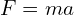.
If we want, we could create an `applyForce` method that takes a force as input and then computes the resulting acceleration based on the body’s mass (which should also be added to the body object fields). At the moment we’re working under the assumption that all the bodies have the same mass.
• Shapes. Our engine only handles circles. If we want to handle arbitrary shapes we’ll need to update all of our constraint handling and collision detection code in order for it to support other shapes.
• Rotation. We didn’t have to handle body rotation because of the very convenient property of circles that they are “rotation invariant” (at least the way we are rendering them now, with uniform color). If we were to add other shapes, this missing feature would be evident as it would result in unrealistic behaviour. For objects to rotate correctly, we would need to implement the rotation equations of motion. Maybe Verlet integration can be used for those as well, but I’m not sure. The only thing I know is they probably involve quaternions. I’ll look into this and maybe keep it for a part II.
• Verlet integration. I’m not an expert in this field at all, so takes this with a grain of salt. As far as I understand, though, this technique is very easy to implement but has issues with robustness and stability that I’m not qualified enough to discuss, so this is something to keep in mind.

## Final demo

Here’s a bigger environment to demo the final engine. This demo is also heavily affected by the p5js `draw` problem we talked about earlier. You might find it in a broken state by now, so I advice you open it in a new tab.

## Conclusions

If you read until here, I hope you had fun and enjoyed the article. Although conciseness was not an objective, it’s interesting to note that the final engine code fits in 100 lines of code!

For me, this kind of work is what made me love programming when I started as a young kid. It has that vibe that only creating something from scratch can give you, and that is often lost when programming professionally. I hope I was able to pass some of that onto you!

Stay well!
Ailef